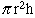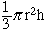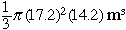My name is Gul and i am a student in grade 9(secondary).ĘI have some math problems. here are the question i am have problem with !! Sand for use on icy roads is stored in a conical pile 14.2 m high and with a base diameter of 34.4 m calculate the volume of the pile if one sander can take 6.9 m of sand, how many sanders can be filled from the pile? thanks alot, Gul Hi Gul, The volume of a cylinder (tin can) is the area of the base times the height. Since the base is a circular disk this gives the area of a cylinder asThis seems quite natural to me, a cylinder is a "stack of disks" so its volume is the area of the disk times the height. The volume of a cone iswhere r is the radius of the base and h is the height. Notice that the volume of the is one-third the volume of the cylinder. Since your cone has height 14.2 m and the radius of the base is 34.4/2 m, the volume isIn your second problem I think that the sanders can each take 6.9 cubic meters of sand. Cheers, Penny Go to Math Central